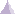# Beam PropertyBeam property is a physical property assigned to a section of a part (1D).

The input and output characteristics are:

• Input:
• Material
• Local Axis (optional)
• Cross-sectional Area
• Moment of inertia (tree values or six values in the case of variable beam)
• Shear Factor (two values )
• Shear Center (two values or two values equal to zero in the case of variable beam)
• Output:

• Point force vector
• Point moment vector

The output characteristics can be expressed at the given positions in the element and in different axis systems:

 Position Axis System Characteristics Center of element Nodes of element Gauss point Global Local Point force vectorPoint moment vector